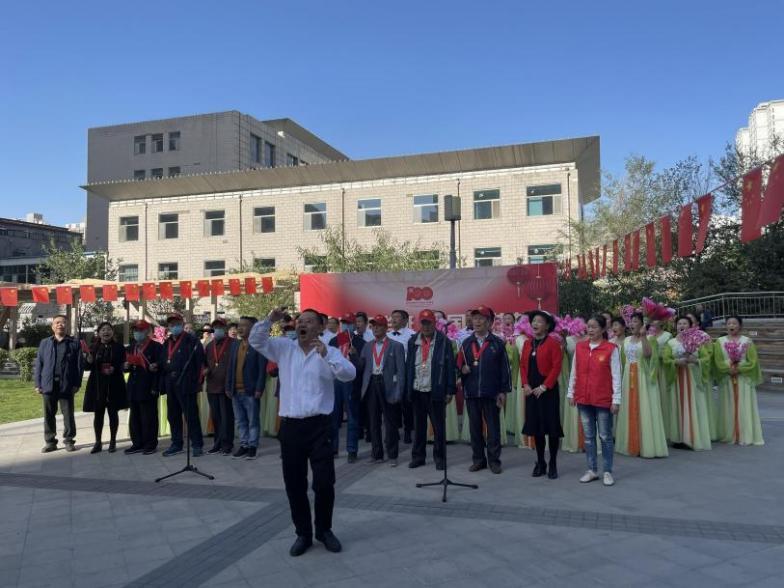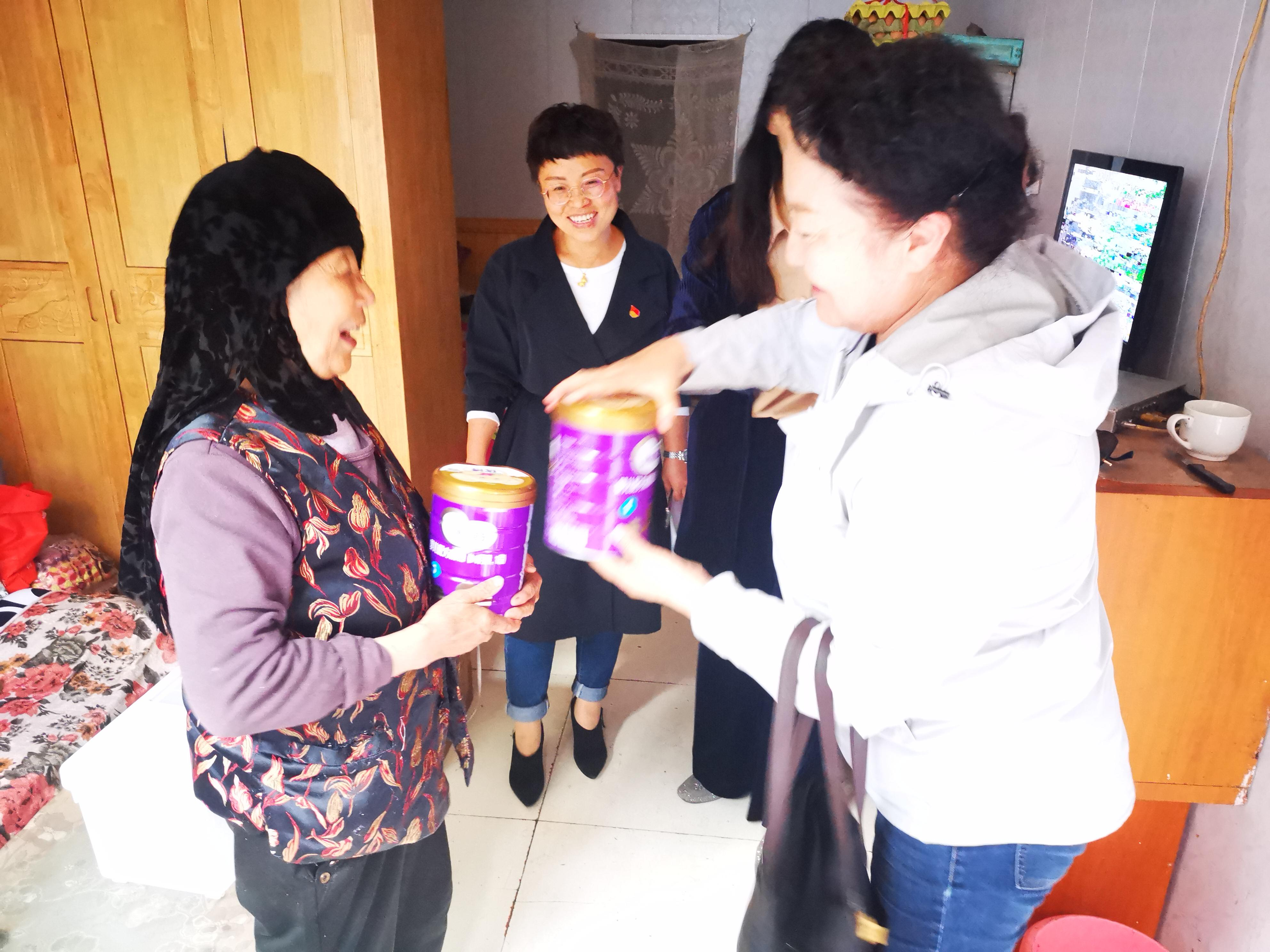# var CalendarData=new Array(20); var madd=new Array(12); var TheDate=new Date(); var tgString="甲乙丙丁戊己庚辛壬癸"; var dzString="子丑寅卯辰巳午未申酉戌亥"; var numString="一二三四五六七八九十"; var monString="正二三四五六七八九十冬腊"; var weekString="日一二三四五六"; var sx="鼠牛虎兔龙蛇马羊猴鸡狗猪"; var cYear; var cMonth; var cDay; var cHour; var cDateString; var DateString; var Browser=navigator.appName; function init() { CalendarData=0x41A95; CalendarData=0xD4A; CalendarData=0xDA5; CalendarData=0x20B55; CalendarData=0x56A; CalendarData=0x7155B; CalendarData=0x25D; CalendarData=0x92D; CalendarData=0x5192B; CalendarData=0xA95; CalendarData=0xB4A; CalendarData=0x416AA; CalendarData=0xAD5; CalendarData=0x90AB5; CalendarData=0x4BA; CalendarData=0xA5B; CalendarData=0x60A57; CalendarData=0x52B; CalendarData=0xA93; CalendarData=0x40E95; madd=0; madd=31; madd=59; madd=90; madd=120; madd=151; madd=181; madd=212; madd=243; madd=273; madd=304; madd=334; } function GetBit(m,n) { return (m>>n)&1; } function e2c() { var total,m,n,k; var isEnd=false; var tmp=TheDate.getYear(); if (tmp<1900) tmp+=1900; total=(tmp-2001)*365 +Math.floor((tmp-2001)/4) +madd[TheDate.getMonth()] +TheDate.getDate() -23; if (TheDate.getYear()%4==0&&TheDate.getMonth()>1) total++; for(m=0;;m++) { k=(CalendarData[m]<0xfff)?11:12; for(n=k;n>=0;n--) { if(total<=29+GetBit(CalendarData[m],n)) { isEnd=true; break; } total=total-29-GetBit(CalendarData[m],n); } if(isEnd)break; } cYear=2001 + m; cMonth=k-n+1; cDay=total; if(k==12) { if(cMonth==Math.floor(CalendarData[m]/0x10000)+1) cMonth=1-cMonth; if(cMonth>Math.floor(CalendarData[m]/0x10000)+1) cMonth--; } cHour=Math.floor((TheDate.getHours()+3)/2); } function GetcDateString() { var tmp=""; tmp+=tgString.charAt((cYear-4)%10); //年干 tmp+=dzString.charAt((cYear-4)%12); //年支 tmp+="年"; tmp+=" "; if(cMonth<1) { tmp+="闰"; tmp+=monString.charAt(-cMonth-1); } else tmp+=monString.charAt(cMonth-1); tmp+="月"; tmp+=(cDay<11)?"初":((cDay<20)?"十":((cDay<30)?"廿":"卅")); if(cDay%10!=0||cDay==10) tmp+=numString.charAt((cDay-1)%10); tmp+=" "; cDateString=tmp; return tmp; } function GetDateString() { var tmp=""; var t1=TheDate.getYear(); if (t1<1900)t1+=1900; tmp+=t1 +"年" +(TheDate.getMonth()+1)+"月" +TheDate.getDate()+"日 " +" 星期"+weekString.charAt(TheDate.getDay()); DateString=tmp; return tmp; } init(); e2c(); GetDateString(); GetcDateString(); setInterval(function(){\$('#cdateRoking').html(GetDateString)},1000); setInterval(function(){\$('#cdate').html(GetcDateString)},1000); 青海省西宁市精神文明建设指导委员会 主办 //无缝滚动 \$(function() { var num = 380; function goLeft() { if (num == -870) { num = 380; } num -= 1; \$(".demo1_top").css({ left: num }) } //设置滚动速度 var timer = setInterval(goLeft, 20); //设置鼠标经过时滚动停止 \$(".top_center2020").hover(function() { clearInterval(timer); }, function() { timer = setInterval(goLeft, 20); }) })西宁文明网>新版>新时代文明实践

# 城中区饮马街街道：缤纷活动献祝福 国庆氛围“别样浓”青ICP备11015360号